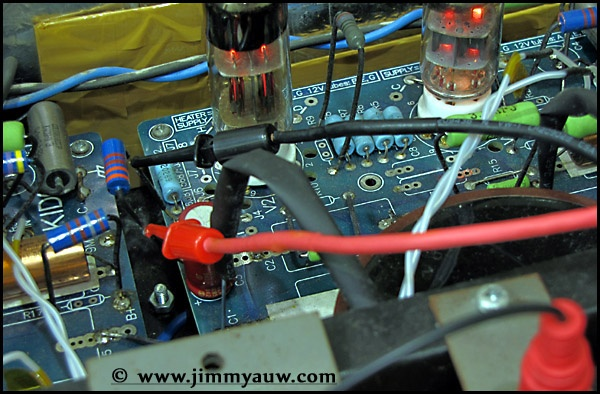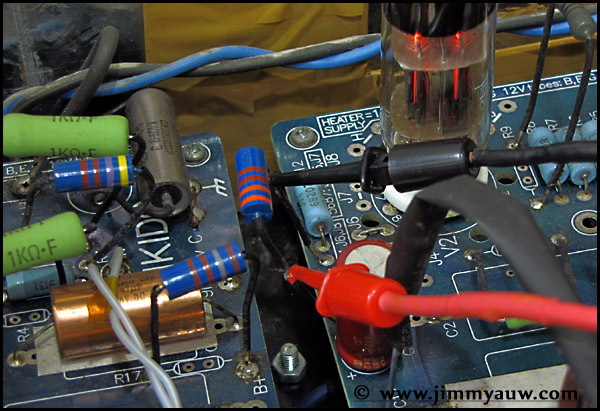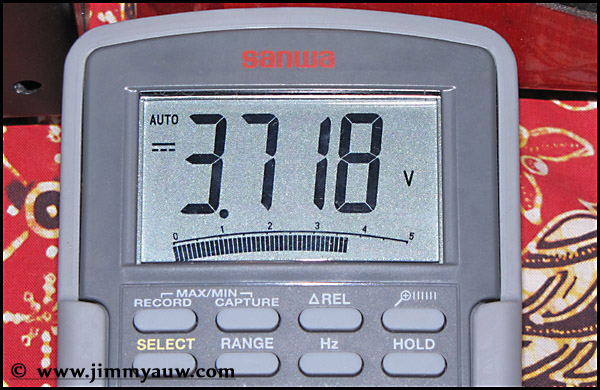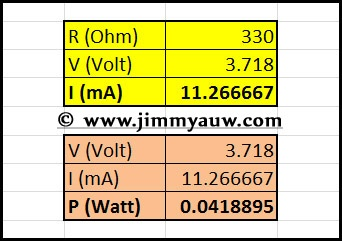Some people send me an email asking about how to measure cathode current and resistor rating. I will show you how to do it and actually this way is not only applicable for cathode resistor (but commonly used for that purpose).

Basically, there is 2 common way to do it. First, put you Ampere meter in series with the resistor. Second, is to measure the voltage on the resistor, then calculate the current based on the Voltage, Current, and Resistance formula (which V=I*R).

For the sake of simplicity, we can opt to use the second method. We cut the shrink from the resistor pins and put the probes there.More detail of the process, we can use hook type probe and put there for longer time to observe more.Value read on the Ampere meter. Since this is only small Pre-Amp, the reading is around 3.7 Volt. Slightly up and down, but we can take the average of the value.We put them on the simple formula (you can always use Excel to simplify your life). Basic formula is V=I*R and P=V*I. V is for Voltage, I for Current, R for Resistance, and P for Power.

As you can see from our sample case. With 330R cathode resistor and 3.718V reading, the current should be around 11mA. Now we know how much current flows on this cathode resistor. Then, we can find the suitable power rating for the resistor. Off course you can always use higher rating for this resistor, but how if you don’t have such option? Example, high end resistor like Shinkoh or AudioNote probably hard to find in higher rating, as they are getting rare. So, what is the safe level?

Base on our calculation, with 3.718V and 11mA current, we can use 0.04W resistor. Well, 0.5W should be common value, 1W should be more than enought, while 2W will be overkill. Some people prefer to spare rating for 3-4x more. So if your calculation produces 0.04W, it’s always comfortable to use 0.5W. If your calculation produces 0.5W, better to go with 2W. Always measure your cathode resistor temperature if you are not sure. More than warm mostly means you have power rating problem. Fix it before it’s too late.